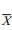# Bootstrap Principle

| Home | | Advanced Mathematics |

## Chapter: Biostatistics for the Health Sciences: Estimating Population Means

The bootstrap principle is very simple.

BOOTSTRAP PRINCIPLE

In Chapter 2, we introduced the concept of bootstrap sampling and told you that it was a nonparametric technique for statistical inference. We also explained the mechanism for generating bootstrap samples and showed how that mechanism is similar to the one used for simple random sampling. In this section, we will de-scribe and use the bootstrap principle to show a simple and straightforward method to generate confidence intervals for population parameters based on the bootstrap samples. Reviewing Chapter 2, the difference between bootstrap sampling and simple random sampling is

1. Instead of sampling from a population, a bootstrap sample is generated by sampling from a sample.

2. The sampling is done with replacement instead of without replacement.

Bootstrap sampling behaves similarly to random sampling in that each bootstrap sample is a sample of size n drawn at random from the empirical distribution Fn, a probability distribution that gives equal weight to each observed data point (i.e., with each draw, each observation has the same chance as any other observation of being the one selected). Similarly, random sampling can be viewed as drawing a sample of size n but from a population distribution F (in which F is an unknown distribution). We are interested in parameters of the distribution that help character-ize the population. In this chapter, we are considering the population mean as the parameter that we would like to know more about.

The bootstrap principle is very simple. We want to draw an inference about the population mean through the sample mean. If we do not make parametric assump-tions (such as assuming the observations have a normal distribution) about the sam-pling distribution of the estimate, we cannot specify the sampling distribution for inference (except approximately through the central limit theorem when the esti-mate is a sample mean).

In constructing confidence intervals, we have considered probability statements about quantities such as Z or t that have the form (– μ)/σor (– μ)/S, where σis the standard deviation or Sis the estimated standard deviation for the sampling distribution (standard error) of the estimated  X. The bootstrap principle attempts to mimic this process of constructing quantities such as Z and t and forming confidence intervals. The sample estimateis replaced by its bootstrap analog*, the mean of a bootstrap sample. The parameter μ is replaced by.

Since the parameter μ is unknown, we cannot actually calculate– μ, but from a bootstrap sample we can calculate* –X. We then approximate the dis-tribution of* –by generating many bootstrap samples and computing many* values. By making the number B of bootstrap replications large, we allow the random generation of bootstrap samples (sometimes called the Monte Carlo method) to approximate as closely as we want the bootstrap distribution of X* –. The histogram of bootstrap samples provides a replacement for the sampling distribution of the Z or t statistic used in confidence interval calculations. The histogram also replaces the normal or t distribution tables that we used in the para-metric approaches.

The idea behind the bootstrap is to approximate the distribution of– μ. If this mimicking process achieves that approximation, then we are able to draw infer-ences about μ. We have no particular reason to believe that the mimicking process actually works.

The bootstrap statistical theory, developed since 1980, shows that under very general conditions, mimicking works as the sample size n becomes large. Other em-pirical evidence from simulation studies has shown that mimicking sometimes works well even with small to moderate sample sizes (10–100). The procedure has been modified and generalized to work for a wide variety of statistical estimation problems.

The bootstrap principle is easy to remember and to apply in general. You mimic the sampling from the population by sampling from the empirical distribution. Wherever the unknown parameters appear in your estimation formulae, you replace them by their estimates from the original sample. Wherever the estimates appear in the formulae, you replace them with their bootstrap estimates. The sample estimates and bootstrap estimates can be thought of as actors. The sample estimates take on the role of the parameters and the bootstrap estimates play the role of the sample es-timates.